Michael Thomas Flanagan's Java Scientific LibraryOutliers Class:     Outlier Detection

Last update: 9 April 2015                                                                                                                             PERMISSION TO USE
Main Page of Michael Thomas Flanagan's Java Scientific Library

This class contains the following outlier detection methods:
• Grubbs’ Test
• Tietjen and Moore Test
• Generalised Extreme Studentised Deviate (ESD) Test
• Dixon's Q Test
Each outlier detection method examines the entered data for deviation from normality, using both a Shapiro-Wilk W test [see Normality Class] and a Gaussian probability plot [see ProbabilityPlot Class], both before and after outlier removal.

import statement:      import flanagan.analysis.Outliers;

SUMMARY OF CONSTRUCTORS AND METHODS

See class ArrayMaths for recasting arrays if the array argument below is not of the type that you require.

 Constructors public Outliers() public Outliers(double[] data) public Outliers(float[] data) public Outliers(long[] data) public Outliers(int[] data) public Outliers(BigDecimal[] data) public Outliers(BigInteger[] data) Read data in from a text file public void readDataFromTextFile() Significance Reset the significance level public void resetSignificance(double significance) Get the significance level public double getSignificance() Output text file Reset the file name public void resetTextFileName(String filename) Supress print to text file public void suppressPrint() Restore print to text file public void restorePrint() Display Probability Plots Suppress display public void suppressDisplay() Restore display public void restoreDisplay() Grubbs’ Test Instant methods public ArrayList

CONSTRUCTOR
public Outliers(double[] data)
public Outliers(float[] data)
public Outliers(long[] data)
public Outliers(int[] data)
public Outliers(BigDecimal[] data)
public Outliers(BigInteger[] data)
public Outliers()
Usage:                      Outliers ol = Outliers(data);
Creates an instance of Outlier. The data to be analysed may be entered via the constructor argument data.
Data of types float, long, int, BigDecimal and BigInteger will be converted to double. The conversion of the latter two will trigger a warning message on possible loss of precision. BigDecimal will trigger an exception if it is too large to convert to double. Data may alternatively be read in from a text file; see Constructor immediately below and also

Usage:                      Outliers ol = Outliers();
This constructor creates an instance of Outlier but requires that the data be read in from a text file; see readDataFromTextFile().

READ IN THE DATA FROM A TEXT FILE
This method allows the data to be analysed to be read in from a text file. On calling this method an Open File dialogue window will be displayed allowing the directories to be searched for the relevant file and for the selection of that file.
The file must be a text file, i.e. a .txt file. The data points must be separated by either one or more spaces, a comma, a semicolon, a colon, a tab or a combination of these. The data is read sequentially along each line of the file. The data may be contained in one or more lines or in a single column. Multiple lines may contain different numbers of points.
This method is best used with the constructor with no data argument (see immediately above). If data has already been entered this method will overwrite the previously entered data.

SIGNIFICANCE
The default value of the significance level used by the outlier detection tests in this class is 0.05 [5%]
This value may be reset to a different user chosen value.

Resetting the significance level
public void resetSignificance(double significance)
Usage:                      ol.resetSignificance(significance);
This method resets the significance level to a user supplied value significance.

Getting the significance level
public double getSignificance()
Usage:                      significance = ol.getSignificance();
This method returns the current significance level.

OUTPUT TO A TEXT FILE
The outlier detection methods all print the results of the outlier search to a text file. The default name of this text file is OutlierDetectionResults.txt. This default file name may be replaced by a name of the user's choice.
The printing to a text file may also be suppressed.

Resetting the file name
public void resetTextFileName(String filename)
Usage:                      ol.resettextFileName(filename);
This method resets the output file name to one supplied by the user via the argument filename.

Suppressing the printing to a text file
public void suppressPrint()
Usage:                     ol.suppressPrint();
This method suppresses the printing of the outlier detection analysis, by instance methods, to a text file.

Restoring the printing to a text file
public void restorePrint()
Usage:                     ol.restorePrint();
This method allows the printing of the outlier detection analysis, by instance methods, to a text file. It need only be called if such printing has been suppressed by calling restorePrint() and now needs restoring.

DISPLAY THE PROBABILITY PLOTS
The outlier detection methods described in this class require the data to be Gaussian [normal] or approximately so. The outlier detection methods all examine the entered data for departures from normality using both a Shapiro-Wilk W test and a Gaussian probability plot [see
ProbabilityPlot Class]. The plot is displayed as a graph of the ordered data versus the corresponding Gaussian order statistic medians. If one or more outliers are detected the data is similarly reexamined for departrures from normality with the outliers removed. Again the graph of the plot is displayed.

The display of the probability plots may also be suppressed.

Suppressing the display of the probability plot/s
public void suppressDisplay()
Usage:                     ol.suppressDisplay();
This method suppresses the display of the probability plot/s by the instance methods.

Restoring the display of the probability plot/s
public void restoreDisplay()
Usage:                     ol.restoreDisplay();
This method restores the display of the probability plot/s by the instance methods. It need only be called if such display has been suppressed by calling restoreDisplay() and now needs restoring.

GRUBBS’ TEST
Grubbs’ test for a single outlier checks the largest standardised residual, G, against a critical Tn value where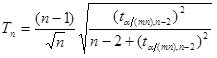n is the number of observations, α is the significance level of the Grubbs’ test [typically 0.05 (5%)] and tα/(nm), n-2 is the student’s t value for a critical probabilty of α/(nm) and n-2 degrees of freedom with m=1 for a one-tailed test and m=2 for a two-tailed test.
An outlier is identified if G>Tn
Grubbs’ test does not behave well in the presence of multiple outliers.
Methods for identifying an upper or lower outlier, a lower outlier or an upper outlier are described below.
Reference:
NIST Engineering Statistics Handbook: Grubbs’ Test for Outliers

Instant methods
Lower or upper outlier
public ArrayList<Object> outlierGrubbs()
Usage:                      ArrayList<Object> outl = ol.outlierGrubbs();
This method tests for the presence of a single outlier, lower or upper, in the data entered via the constructor, at the default significance level. An outlier is identified if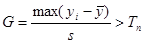where ȳ is the sample data mean and s is the sample data standard deviation.
The output ArrayList contains the elements:
 element 0 (boolean) true if an outlier identified, false if not element 1 (double) the value of the outlier if an outlier identified, NaN if not element 2 (int) the index of the outlier if an outlier identified, -1 if not element 3 (double[]) the data array with the outlier removed, the original data array if no outlier identified element 4 (double) Grubbs’ G value element 5 (double) Grubbs’ Tn value element 6 (double) significance level used element 7 (int) number of data points element 8 (double) gradient of a Gaussian probability plot of the entered data element 9 (double) intercept of a Gaussian probability plot of the entered data element 10 (double) correlation coefficient, r, of a Gaussian probability plot of the entered data element 11 (double) critical value for the correlation coefficient, r, of a Gaussian probability plot of the entered data at the significance level returned as element 6 element 12 (double) gradient of a Gaussian probability plot of the data with the outlier removed if outlier found, NaN otherwise element 13 (double) intercept of a Gaussian probability plot of the data with the outlier removed if outlier found, NaN otherwise element 14 (double) correlation coefficient, r, of a Gaussian probability plot of the data with the outlier removed if outlier found, NaN otherwise element 15 (double) critical value for the correlation coefficient, r, of a Gaussian probability plot of the data with the outlier removed at the significance level returned as element 6 if outlier found, NaN otherwise element 16 (double) Shapiro-Wilk W value for the entered data element 17 (double) Critical value of the Shapiro-Wilk W value for the entered data at at the significance level returned as element 6 element 18 (double) Shapiro-Wilk p value for the entered data element 19 (double) Shapiro-Wilk W value for the data with the outlier removed if outlier found, NaN otherwise element 20 (double) Critical value of the Shapiro-Wilk W value for the data with the outlier removed at at the significance level returned as element 6 if outlier found, NaN otherwise element 21 (double) Shapiro-Wilk p value for the data with the outlier removed if outlier found, NaN otherwise
This method also prints the above analysis to a text file, OutlierDetectionResults.txt. A typical output text file is shown below: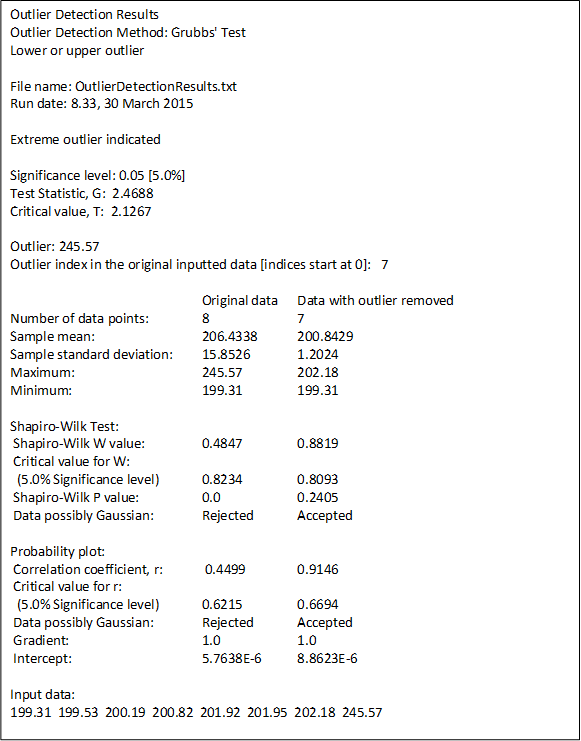[Data from Tietjen and Moore (August 1972), Some Grubbs-Type Statistics for the Detection of Outliers, Technometrics, 14(3), pp. 583-597]
See above for methods allowing a change of file name and for suppressing this print to a text file.

Lower outlier
public ArrayList<Object> lowerOutlierGrubbs()
Usage:                      ArrayList<Object> outl = ol.lowerOutlierGrubbs();
This method tests for the presence of a single lower outlier in the data entered via the constructor, at the default significance level. An outlier is identified if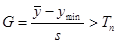where ymin is the minimum data value, ȳ is the sample data mean and s is the sample data standard deviation.
This method also prints an analysis to a text file, OutlierDetectionResults.txt.
The output array and text file are as described above for the outlierGrubbs() method.

Upper outlier
public ArrayList<Object> upperOutlierGrubbs()
Usage:                      ArrayList<Object> outl = ol.upperOutlierGrubbs();
This method tests for the presence of a single upper outlier in the data entered via the constructor, at the default significance level. An outlier is identified if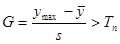where ymax is the maximum data value, ȳ is the sample data mean and s is the sample data standard deviation.
This method also prints an analysis to a text file, OutlierDetectionResults.txt.
The output array and text file are as described above for the outlierGrubbs(significance) method.

Grubbs’ Tn for a One-Tailed Test
public double getGrubbsOneTailedCriticalT()
Usage:                      grubbsT = ol.getGrubbsOneTailedCriticalT();
This method returns, for n data points where n is the length of the data array entered via the constructor, for the default significance level, α, and for a one-tailed test, the Tn value wheren is the number of observations, α is the significance level of the Grubbs’ test [typically 0.05 (5%)] and tα/(nm), n-2 is the student’s t value for a critical probabilty of α/(nm) and n-2 degrees of freedom with m=1.

Grubbs’ Tn for a Two-Tailed Test
public double getGrubbsTwoTailedCriticalT()
Usage:                      grubbsT = Stat.getGrubbsTwoTailedCriticalT();
This method returns, for n data points where n is the length of the data array entered via the constructor, for the default significance level, α, and for a two-tailed test, the Tn value wheren is the number of observations, α is the significance level of the Grubbs’ test [typically 0.05 (5%)] and tα/(nm), n-2 is the student’s t value for a critical probabilty of α/(nm) and n-2 degrees of freedom with m=2.

Static methods
Lower or upper outlier
public static ArrayList<Object> outlierGrubbs(double[] data)
Usage:                      ArrayList<Object> outl = Stat.outlierGrubbs(data);
This method tests for the presence of a single outlier, lower or upper, in the data entered via the argument data, at the default significance level An outlier is identified ifThis method also prints an analysis to a text file, OutlierDetectionResults.txt.
The output array and text file are as described above for the outlierGrubbs() method.

Lower outlier
public static ArrayList<Object> lowerOutlierGrubbs(double[] data)
Usage:                      ArrayList<Object> outl = Stat.lowerOutlierGrubbs(data);
This method tests for the presence of a single lower outlier in the data entered via the argument data, at the default significance level An outlier is identified ifThis method also prints an analysis to a text file, OutlierDetectionResults.txt.
The output array and text file are as described above for the outlierGrubbs() method.

Upper outlier
public static ArrayList<Object> upperOutlierGrubbs(double[] data)
Usage:                      ArrayList<Object> outl = Stat.upperOutlierGrubbs(data);
This method tests for the presence of a single upper outlier in the data entered via the argument data, at the default significance level An outlier is identified ifThis method also prints an analysis to a text file, OutlierDetectionResults.txt.
The output array and text file are as described above for the outlierGrubbs() method.

Grubbs’ Tn for a One-Tailed Distribution Test
public static double getGrubbsOneTailedCriticalT(double significance, int nObservations)
Usage:                      grubbsT = Stat.getGrubbsOneTailedCriticalT(significance, nObservations);
This method returns, for n [nObservations], a significance level of α [significance] and a one-tailed test, the Tn value wheren is the number of observations, α is the significance level of the Grubbs’ test [typically 0.05 (5%)] and tα/(nm), n-2 is the student’s t value for a critical probabilty of α/(nm) and n-2 degrees of freedom with m=1.

Grubbs’ Tn for a Two-Tailed Distribution Test
public static double getGrubbsTwoTailedCriticalT(double significance, int nObservations)
Usage:                      grubbsT = Stat.getGrubbsTwoTailedCriticalT(significance, nObservations);
This method returns, for n [nObservations], a significance level of α [significance] and a two-tailed test, the Tn value wheren is the number of observations, α is the significance level of the Grubbs’ test [typically 0.05 (5%)] and tα/(nm), n-2 is the student’s t value for a critical probabilty of α/(nm) and n-2 degrees of freedom with m=2.

TIETJEN-MOORE TEST
This test is a generalisation of the Grubbs’ Test for more than one outlier. However the number of outliers must be specified.
The critical value of the test statistic is obtained from several, 10000 by default, simulations using randomly generated normal distributions of n points where n is the number of points in the analyzed data. 10000 smulations gives a critical value with an approximate error of 1%. The default value, 10000, may be altered. Methods for identifying upper or lower outliers, lower outliers or upper outliers are described below.
Reference:
NIST Engineering Statistics Handbook: Tietjen-Moore Test for Outliers

Instant methods
Lower or upper outliers
public ArrayList<Object> outliersTietjenMoore(int nOutliers)
Usage:                      ArrayList<Object> outl = ol.outliersTietjenMoore(nOutliers);
This method tests for the presence of k [nOutliers] outliers, lower or upper, in the data entered via the constructor, at the default
significance level. An outlier is identified if Lk < Lcrit where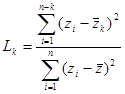z are the n entered data points, y, arranged in ascending order of the absolute values of their residuals, r, where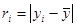where ȳ and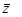are both the sample data mean and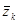is the mean of the sample data with the largest k z points removed. Lcrit is determined by simulation. The simulation is performed by generating a standard normal random sample of size n and computing the Lk statistic. This simulation is performed m times. The default value of m is 10000. This default value may be altered [see Simulation number]. The value of Lcrit is obtained from this reference distribution. The value of the test statistic L lies between zero and one. In the absence of outliers the test statistic approaches 1. In the presence of outliers the test statistic approaches zero. The test is a lower, one-tailed test.

The output ArrayList contains the elements:
 element 0 (boolean) true if outliers identified, false if not element 1 (double[]) the value of the data points chosen as potential outliers [outliers if element 0 is true] element 2 (int[]) the indices of the potential/identified outliers. element 3 (double[]) the data array with the outliers removed, the original data array if no outliers identified element 4 (double) data sample Lk value element 5 (double) simulation Lcrit value element 6 (double) significance level used element 7 (double) significance level at which Lk = Lcrit element 8 (int) number of data points element 9 (int) number of outliers/possible outliers element 10 (double) gradient of a Gaussian probability plot of the entered data element 11 (double) intercept of a Gaussian probability plot of the entered data element 12 (double) correlation coefficient, r, of a Gaussian probability plot of the entered data/td> element 13 (double) critical value for the correlation coefficient, r, of a Gaussian probability plot of the entered data at the significance level returned as element 6 element 14 (double) gradient of a Gaussian probability plot of the data with the outlier removed if outlier/s found, NaN otherwise element 15 (double) intercept of a Gaussian probability plot of the data with the outlier removed if outlier/s found, NaN otherwise element 16 (double) correlation coefficient, r, of a Gaussian probability plot of the data with the outlier removed if outlier/s found, NaN otherwise element 17 (double) critical value for the correlation coefficient, r, of a Gaussian probability plot of the data with the outlier removed at the significance level returned as element 6 if outlier/s found, NaN otherwise element 18 (double) Shapiro-Wilk W value for the entered data element 19 (double) Critical value of the Shapiro-Wilk W value for the entered data at at the significance level returned as element 6 element 20 (double) Shapiro-Wilk p value for the entered data element 21 (double) Shapiro-Wilk W value for the data with the outlier removed if outlier found, NaN otherwise element 22 (double) Critical value of the Shapiro-Wilk W value for the data with the outlier removed at at the significance level returned as element 6 if outlier found, NaN otherwise element 23 (double) Shapiro-Wilk p value for the data with the outlier removed if outlier found, NaN otherwise

This method also prints the above analysis to a text file, OutlierDetectionResults.txt. A typical output text file is shown below: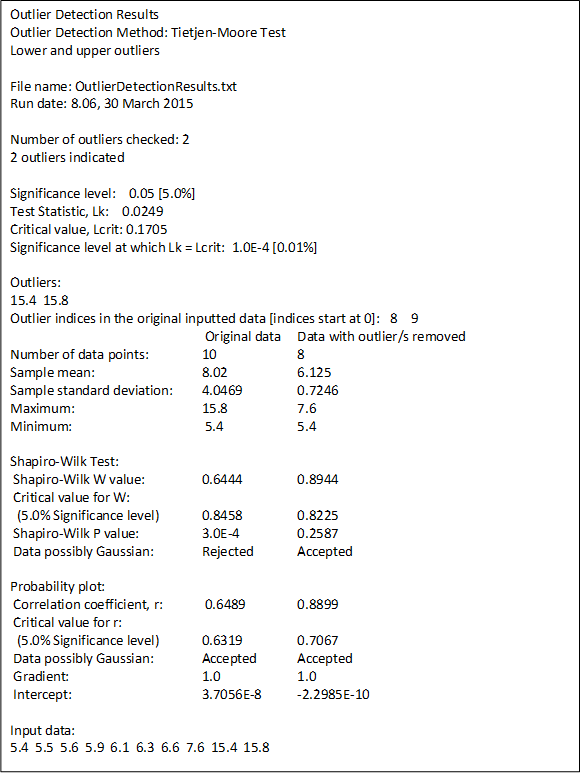[Data taken from Grubbs, Frank (1950), Sample Criteria for Testing Outlying Observations, Annals of Mathematical Statistics, 21(1) pp. 27-58.]
See above for methods allowing a change of file name and for suppressing this print to a text file.

Lower outliers
public ArrayList<Object> lowerOutliersTietjenMoore(int nOutliers)
Usage:                      ArrayList<Object> outl = ol.lowerOutliersTietjenMoore(nOutliers);
This method tests for the presence of k [nOutliers] lower outliers in the data entered via the constructor, at the default significance level. An outlier is identified if Lk < Lcrit where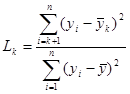y are the n entered data points arranged in descending order, ȳ is the sample data mean and ȳk is the mean of the sample data with the smallest k points removed.
Lcrit is determined as described above for the method outliersTietjenMoore(nOutliers).
This method also prints an analysis to a text file, OutlierDetectionResults.txt.
The contents of the output array and the text file are also as described above for the method outliersTietjenMoore(nOutliers).

Upper outliers
public ArrayList<Object> upperOutliersTietjenMoore(int nOutliers)
Usage:                      ArrayList<Object> outl = ol.upperOutliersTietjenMoore(nOutliers);
This method tests for the presence of k [nOutliers] upper outliers in the data entered via the constructor, at the default significance level. An outlier is identified if Lk < Lcrit where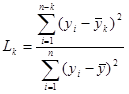y are the n entered data points arranged in ascending order, ȳ is the sample data mean and ȳk is the mean of the sample data with the largest k points removed.
Lcrit is determined as described above for the method outliersTietjenMoore(nOutliers).
This method also prints an analysis to a text file, OutlierDetectionResults.txt.
The contents of the output array and of the text file are also as described above for the method outliersTietjenMoore(nOutliers).

Critical L [Lcrit] Values
public double getTietjenMooreCriticalL(int nOutliers)
Usage:                      lcrit = ol.getTietjenMooreCriticalL(nOutliers);
This method returns the value Lcrit for nOutliers upper or lower outliers at the default significance level.

public double getTietjenMooreLowerCriticalL(int nOutliers)
Usage:                      lcrit = ol.getTietjenMooreLowerCriticalL(nOutliers);
This method returns the value Lcrit for nOutliers lower outliers at the default significance level.

public double getTietjenMooreUpperCriticalL(int nOutliers)
Usage:                      lcrit = ol.getTietjenMooreUpperCriticalL(nOutliers);
This method returns the value Lcrit for nOutliers upper outliers at the default significance level.

Number of simulations
public void resetNumberTietjenMooreSimulations(int nSimulations)
Usage:                      ol.resetNumberTietjenMooreSimulations(nSimulations);
This method resets the value of the number of simulations [nSimulations] used in the calculation of Lcrit. See
method outliersTietjenMoore(nOutliers) for details of this calculation. The default value of the number of simulations is 10000.

public int getNumberTietjenMooreSimulations()
Usage:                      nSimulations = ol.getNumberTietjenMooreSimulations();
This method returns the value of the number of simulations [nSimulations] used in the calculation of Lcrit. See method outliersTietjenMoore(nOutliers) for details of this calculation. The default value of the number of simulations is 10000.

Static methods
Lower or upper outliers
public static ArrayList<Object> outliersTietjenMoore(double[] data, int nOutliers)
Usage:                      ArrayList<Object> outl = Outliers.outliersTietjenMoore(data, nOutliers);
This method tests for the presence of k [nOutliers] outliers, lower or upper, in the data entered via the argument data, at the default significance level. An outlier is identified if Lk < Lcrit wherez are the n entered data points, y, arranged in ascending order of the absolute values of their residuals, r, wherewhere ȳ andare both the sample data mean andis the mean of the sample data with the largest k z points removed.
Lcrit is determined as described above for the method outliersTietjenMoore(nOutliers).
This method also prints an analysis to a text file, OutlierDetectionResults.txt.
The contents of the output array and of the text file are also as described above for the method outliersTietjenMoore(nOutliers).

Lower outliers
public static ArrayList<Object> lowerOutliersTietjenMoore(double[] data, int nOutliers)
Usage:                      ArrayList<Object> outl = Outliers.lowerOutliersTietjenMoore(data, nOutliers);
This method tests for the presence of k [nOutliers] lower outliers in the data entered via the argument data, at the default significance level. An outlier is identified if Lk < Lcrit wherey are the n entered data points arranged in descending order, ȳ is the sample data mean and ȳk is the mean of the sample data with the smallest k points removed.
Lcrit is determined as described above for the method method outliersTietjenMoore(nOutliers).
This method also prints an analysis to a text file, OutlierDetectionResults.txt.
The contents of the output array and of the text file are also as described above for the method outliersTietjenMoore(nOutliers).

Upper outliers
public ArrayList<Object> upperOutliersTietjenMoore(double[] data, int nOutliers)
Usage:                      ArrayList<Object> outl = Outliers.upperOutliersTietjenMoore(data, nOutliers);
This method tests for the presence of k [nOutliers] upper outliers in the data entered via the argument data, at the default significance level. An outlier is identified if Lk < Lcrit wherey are the n entered data points arranged in ascending order, ȳ is the sample data mean and ȳk is the mean of the sample data with the largest k points removed.
Lcrit is determined as described above for the method outliersTietjenMoore(nOutliers).
This method also prints an analysis to a text file, OutlierDetectionResults.txt.
The contents of the output array and of the text file are also as described above for the method outliersTietjenMoore(nOutliers).

Critical L [Lcrit] Values
public static double getTietjenMooreCriticalL(int nOutliers, int nObservations, int nSimultions, double significance)
Usage:                      lcrit = Outliers.getTietjenMooreCriticalL(nOutliers, nObservations, nSimulations, significance);
This method returns the value Lcrit for nOutliers upper or lower outliers at the entered significance level, significance, for nObservations data points and nSimulations simulations in the calculation of Lcrit.

public static double getTietjenMooreCriticalL(int nOutliers, int nObservations, double significance)
Usage:                      lcrit = Outliers.getTietjenMooreCriticalL(nOutliers, nObservations, significance);
This method returns the value Lcrit for nOutliers upper or lower outliers at the entered significance level, significance, for nObservations data points and 10000 simulations in the calculation of Lcrit.

public static double getTietjenMooreLowerCriticalL(int nOutliers, int nObservations, int nSimultions, double significance)
Usage:                      lcrit = Outliers.getTietjenMooreLowerCriticalL(nOutliers, nObservations, nSimulations, significance);
This method returns the value Lcrit for nOutliers lower outliers at the entered significance level, significance, for nObservations data points and nSimulations simulations in the calculation of Lcrit.

public static double getTietjenMooreLowerCriticalL(int nOutliers, int nObservations, double significance)
Usage:                      lcrit = Outliers.getTietjenMooreLowerCriticalL(nOutliers, nObservations, significance);
This method returns the value Lcrit for nOutliers lower outliers at the entered significance level, significance, for nObservations data points and 10000 simulations in the calculation of Lcrit.

public static double getTietjenMooreUpperCriticalL(int nOutliers, int nObservations, int nSimultions, double significance)
Usage:                      lcrit = Outliers.getTietjenMooreUpperCriticalL(nOutliers, nObservations, nSimulations, significance);
This method returns the value Lcrit for nOutliers upper outliers at the entered significance level, significance, for nObservations data points and nSimulations simulations in the calculation of Lcrit.

public static double getTietjenMooreUpperCriticalL(int nOutliers, int nObservations, double significance)
Usage:                      lcrit = Outliers.getTietjenMooreUpperCriticalL(nOutliers, nObservations, significance);
This method returns the value Lcrit for nOutliers upper outliers at the entered significance level, significance, for nObservations data points and 10000 simulations in the calculation of Lcrit.

GENERALISED ESD (EXTREME STUDENTISED DEVIATE) TEST
This test is a generalisation of the Grubbs’ Test for more than one outlier.
Reference:
NIST Engineering Statistics Handbook: Generalized ESD Test for Outliers

Instant methods
Lower or upper outliers
public ArrayList<Object> outliersESD(int nPossibleOutliers)
Usage:                      ArrayList<Object> outl = ol.outliersESD(nPossibleOutliers);
This method tests for the presence of up to nPossibleOutliers upper or lower outliers in the data entered via the constructor, at the default
significance level.
The statistic Ri is first calculated for the whole sample, i.e. i = 1, where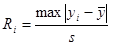ȳ is the sample data mean and s is the sample standard deviation. This statistic is then compared with a critical value, λi, calculated, with i = 1, as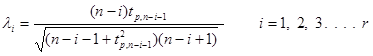where n is the number of data points, tp, n-i-1 is the student’s t value for a critical probabilty of p and n-i-1 degrees of freedom,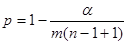α is the significance level of this ESD test and m=2 [two-tailed test].
The point of maximum R is removed and the above calcultion and comparison made with the remaining points and i = 2.
This procedure is then repeated until the estimated number of outliers, r [enterted as argument nPossibleOutliers], points have been removed.
The number of outliers is the highest value of i for which Ri>λi.
See example of output find below for an example.

The output ArrayList contains the elements:
 element 0 (boolean) true if outliers identified, false if not element 1 (double[]) the values of the data points indicated as outliers; null if no outliers indicated element 2 (int[]) the indices of the identified outliers; null if no outliers indicated element 3 (double[]) the data array with the outliers removed, the original data array if no outliers identified element 4 (double) Test Statistics Ri value element 5 (double) Critical Values λi element 6 (double) true or false values for Ri > λi element 7 (double) data values for the maximum ESDs element 8 (double) significance level used element 9 (int) number of data points element 10 (int) number of points tested as possible outliers element 11 (double) gradient of a Gaussian probability plot of the entered data element 12 (double) intercept of a Gaussian probability plot of the entered data element 13 (double) correlation coefficient, r, of a Gaussian probability plot of the entered data element 14 (double) critical value for the correlation coefficient, r, of a Gaussian probability plot of the entered data at the significance level returned as element 6 element 15 (double) gradient of a Gaussian probability plot of the data with the outlier removed if outlier/s found, NaN otherwise element 16 (double) intercept of a Gaussian probability plot of the data with the outlier removed if outlier/s found, NaN otherwise element 17 (double) correlation coefficient, r, of a Gaussian probability plot of the data with the outlier/s removed if outlier found, NaN otherwise element 18 (double) critical value for the correlation coefficient, r, of a Gaussian probability plot of the data with the outlier removed at the significance level returned as element 6 if outlier found, NaN otherwise element 19 (double) Shapiro-Wilk W value for the entered data element 20 (double) Critical value of the Shapiro-Wilk W value for the entered data at at the significance level returned as element 6 element 21 (double) Shapiro-Wilk p value for the entered data element 22 (double) Shapiro-Wilk W value for the data with the outlier removed if outlier found, NaN otherwise element 23 (double) Critical value of the Shapiro-Wilk W value for the data with the outlier removed at at the significance level returned as element 6 if outlier found, NaN otherwise element 24 (double) Shapiro-Wilk p value for the data with the outlier removed if outlier found, NaN otherwise

This method also prints the above analysis to a text file, OutlierDetectionResults.txt. A typical output text file, in which the top 10 successive maximum ESDs were examined as possible outliers, is shown below: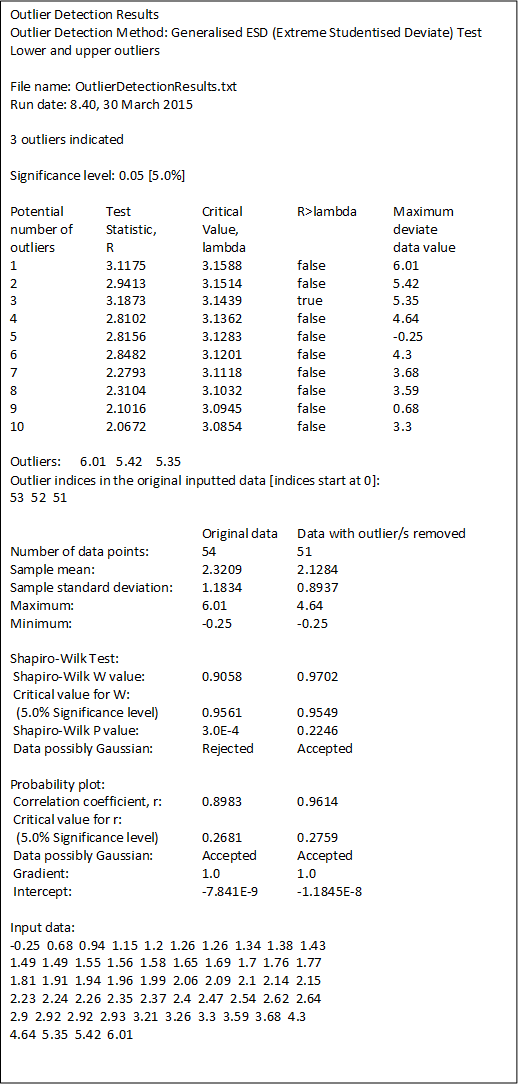[Data taken from Rosner, Bernard (May 1983), Percentage Points for a Generalized ESD Many-Outlier Procedure,Technometrics, 25(2), pp. 165-172.]
See above for methods allowing a change of file name and for suppressing this print to a text file.

Static methods
Lower or upper outliers
public static ArrayList<Object> outliersESD(double[] data, publicint nOutliers)
Usage:                      ArrayList<Object> outl = Outliers.outliersESD(data, nOutliers);
This method tests for the presence of up to nOutliers upper or lower outliers in the data entered via the argument data, at the default significance level.
This method also prints an analysis to a text file, OutlierDetectionResults.txt.
The output array and text file are as described above for the outlierESD(nOutliers) method.

Critical values, λi
public static double[] getESDlambdas(intrPoints, int nPoints, double significance)
Usage:                      lambdas = Outliers.getESDlambdas(rPoints, nPoints, significance);
This method returns the r [rPoints] values of λifor n [nPoints] data points, a significance level, α, entered as the argument significance and m = 2 [two-tailed test].
See esdOutliers(nPossibleOutliers) above for a full description of λi.

DIXON'S Q TEST
This test is for a single extreme outlier but options are available for ignoring data points below the extreme value being tested and at the other extreme of the ordered data if these may be causing masking.
The critical value of the test statistic is obtained from several, 10000 by default, simulations using randomly generated normal distributions of n points where n is the number of points in the analyzed data. 10000 smulations gives a critical value with an approximate error of 1%. The default value, 10000, may be altered.
Methods for identifying upper or lower outliers, lower outliers or upper outliers are described below.

Instance methods
Test for a single lower or upper outlier with an option of ignoring some points
public ArrayList<Object> outlierDixon(int a, int b)
Usage:                      ArrayList<Object> outl = ol.outlierDixon(a, b);
This method tests for a single lower or upper outlier in the data entered via a constructor at the
default significance level. An outlier is identified if
rab  >  Qcrit
where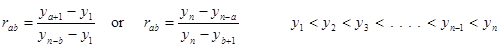and y is the data ordered, by this method, as ascending values. This ratio allows for the ignoring of some data points, i.e. the test is for y1 avoiding points y2, y3 . . . ya [a>0] and points yn, yn-1 . . . yn-b+1 [b>0] if the single extreme point is the lowest point or yn-1, yn-2 . . . yn-a+1 [a>0] and points y1, y2 . . . yb [b>0] if it is the highest point.
The values of a and b are entered as the arguments a and b. The critical value, Qcrit is determined by simulation. The simulation is performed by generating a standard normal random sample of size n and computing the rab statistic. This simulation is performed m times. The default value of m is 10000. This default value may be altered [see Simulation number]. The value of Qcrit is obtained from this reference distribution. No points are ignored if a = 1 and b = 0, i.e. if r10 is calculated; using r10 gives a simple search for a single lower or upper outlier using all the data and has its own method described below under outlierDixon().

The output ArrayList contains the elements:
 element 0 (boolean) true if outliers identified, false if not element 1 (double) the value of outlier; NaN if no outlier found element 2 (int) the index of the outlier point; -1 if no outlier found. element 3 (double[]) the data array with the outliers removed, the original data array if no outliers identified element 4 (double) data sample rab value element 5 (double) simulation Qcrit value element 6 (double) significance level used element 7 (int) number of data points element 8 (int) value of a in rab element 9 (int) value of b in rab element 10 (int) number of points ignored element 11 (double[]) values of ignored points; null if no points ignored element 12 (double) gradient of a Gaussian probability plot of the entered data element 13 (double) intercept of a Gaussian probability plot of the entered data element 14 (double) correlation coefficient, r, of a Gaussian probability plot of the entered data element 15 (double) critical value for the correlation coefficient, r, of a Gaussian probability plot of the entered data at the significance level returned as element 6 element 16 (double) gradient of a Gaussian probability plot of the data with the outlier removed if outlier found, NaN otherwise element 17 (double) intercept of a Gaussian probability plot of the data with the outlier removed if outlier found, NaN otherwise element 18 (double) correlation coefficient, r, of a Gaussian probability plot of the data with the outlier removed if outlier found, NaN otherwise element 19 (double) critical value for the correlation coefficient, r, of a Gaussian probability plot of the data with the outlier removed at the significance level returned as element 6 if outlier found, NaN otherwise element 20 (double) Shapiro-Wilk W value for the entered data element 21 (double) Critical value of the Shapiro-Wilk W value for the entered data at at the significance level returned as element 6 element 22 (double) Shapiro-Wilk p value for the entered data element 23 (double) Shapiro-Wilk W value for the data with the outlier removed if outlier found, NaN otherwise element 24 (double) Critical value of the Shapiro-Wilk W value for the data with the outlier removed at at the significance level returned as element 6 if outlier found, NaN otherwise element 25 (double) Shapiro-Wilk p value for the data with the outlier removed if outlier found, NaN otherwise
This method also prints the above analysis to a text file, OutlierDetectionResults.txt. A typical output text file, for rab = r10, i.e. a = 1 and b = 2, point n-1 is ignored in the case of an upper outlier which is the case, is shown below: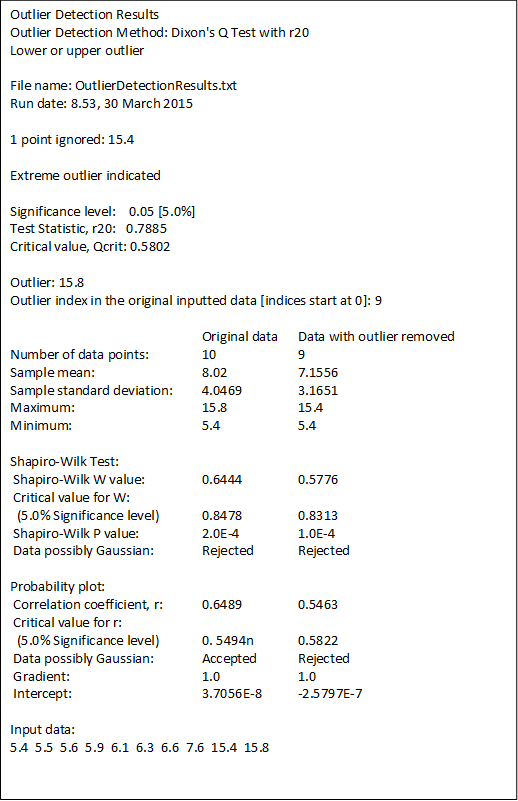See above for methods allowing a change of file name and for suppressing this print to a text file.

Test for a single lower or upper outlier with no points ignored
public ArrayList<Object> outlierDixon()
Usage:                      ArrayList<Object> outl = ol.outlierDixon();
This method tests for a single lower or upper outlier in the data entered via a constructor at the
default significance level. An outlier is identified if
r10  >  Qcrit
where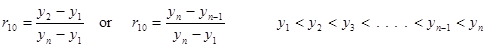and y is the data ordered, by this method, as ascending values. No points are ignored. Qcrit is calculated as described in outlierDixon(a,b) above.
The output array and outputted text file are also as described under outlierDixon(a,b).

Test for a single lower outlier with an option of ignoring some points
public ArrayList<Object> lowerOutlierDixon(int a, int b)
Usage:                      ArrayList<Object> outl = ol.lowerOutlierDixon(a, b);
This method tests for a single lower outlier in the data entered via a constructor at the default significance level. An outlier is identified if
rab  >  Qcrit
where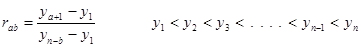and y is the data ordered, by this method, as ascending values. This ratio allows for the ignoring of some data points, i.e. the test is for y1 avoiding points y2, y3 . . . ya [a>0] and points yn, yn-1 . . . yn-b+1 [b>0].
The values of a and b are entered as the arguments a and b. The critical value, Qcrit is determined by simulation. The simulation is performed by generating a standard normal random sample of size n and computing the rab statistic. This simulation is performed m times. The default value of m is 10000. This default value may be altered [see Simulation number]. The value of Qcrit is obtained from this reference distribution.
No points are ignored if a = 1 and b = 0, i.e. if r10 is calculated; using r10 gives a simple search for a single lower outlier using all the data and has its own method described below under lowerOutlierDixon().
The output array and outputted text file are as described under outlierDixon(a,b).

Test for a single lower outlier with no points ignored
public ArrayList<Object> lowerOutlierDixon()
Usage:                      ArrayList<Object> outl = ol.lowerOutlierDixon();
This method tests for a single lower outlier in the data entered via a constructor at the default significance level. An outlier is identified if
r10  >  Qcrit
where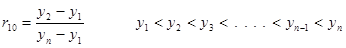and y is the data ordered, by this method, as ascending values. No points are ignored. Qcrit is calculated as described in outlierDixon(a,b) above.
The output array and outputted text file are also as described under outlierDixon(a,b).

Test for a single upper outlier upper with an option of ignoring some points
public ArrayList<Object> upperOutlierDixon(int a, int b)
Usage:                      ArrayList<Object> outl = ol.upperOutlierDixon(a, b);
This method tests for a single upper outlier in the data entered via a constructor at the default significance level. An outlier is identified if
rab  >  Qcrit
where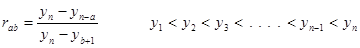and y is the data ordered, by this method, as ascending values. This ratio allows for the ignoring of some data points, i.e. the test is for yn-1, yn-2 . . . yn-a+1 [a>0] and points y1, y2 . . . yb [b>0].
The values of a and b are entered as the arguments a and b. The critical value, Qcrit is determined by simulation. The simulation is performed by generating a standard normal random sample of size n and computing the rab statistic. This simulation is performed m times. The default value of m is 10000. This default value may be altered [see Simulation number]. The value of Qcrit is obtained from this reference distribution. No points are ignored if a = 1 and b = 0, i.e. if r10 is calculated; using r10 gives a simple search for a single upper outlier using all the data and has its own method described below under upperOutlierDixon().
The output array and outputted text file are as described under outlierDixon(a,b).

Test for a single upper outlier with no points ignored
public ArrayList<Object> upperOutlierDixon()
Usage:                      ArrayList<Object> outl = ol.upperOutlierDixon();
This method tests for a single lower outlier in the data entered via a constructor at the default significance level. An outlier is identified if
r10  >  Qcrit
where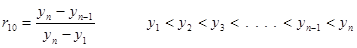and y is the data ordered, by this method, as ascending values. No points are ignored. Qcrit is calculated as described in outlierDixon(a,b) above.
The output array and outputted text file are also as described under outlierDixon(a,b).

Number of simulations
public void resetNumberDixonSimulations(int nSimulations)
Usage:                      ol.resetNumberDixonSimulations(nSimulations);
This method resets the value of the number of simulations [nSimulations] used in the calculation of Qcrit. See
method outliersDixon(a,b) for details of this calculation. The default value of the number of simulations is 10000.

public int getNumberDixonSimulations()
Usage:                      nSimulations = ol.getNumberDixonSimulations();
This method returns the value of the number of simulations [nSimulations] used in the calculation of Qsub>crit. See method outliersDixon(a, b) for details of this calculation. The default value of the number of simulations is 10000.

Static methods
Test for a single lower or upper outlier with an option of ignoring some points
public static ArrayList<Object> outlierDixon(double[] data, int a, int b)
Usage:                      ArrayList<Object> outl = outliers.outlierDixon(data, a, b);
This method tests for a single lower or upper outlier in the data entered via the argument data at a significance level of 0.05. An outlier is identified if
rab  >  Qcrit
whereand y is the data ordered, by this method, as ascending values. The number of simulations used in calculating Qcrit is 10000. The method is, otherwise, the same as the corresponding instance method outlierDixon(a, b).

Test for a single lower or upper outlier with no points ignored
public static ArrayList<Object> outlierDixon(double[] data)
Usage:                      ArrayList<Object> outl = outliers.outlierDixon();
This method tests for a single lower or upper outlier in the data entered via the argument data at significance level of 0.05. An outlier is identified if
r10  >  Qcrit
whereand y is the data ordered, by this method, as ascending values. The number of simulations used in calculating Qcrit is 10000. The method is, otherwise, the same as the corresponding instance method outlierDixon().

Test for a single lower outlier with an option of ignoring some points
public static ArrayList<Object> lowerOutlierDixon(double[] data, int a, int b)
Usage:                      ArrayList<Object> outl = outliers.lowerOutlierDixon(data, a, b);
This method tests for a single lower outlier in the data entered via the argument data at a significance level of 0.05. An outlier is identified if
rab  >  Qcrit
whereand y is the data ordered, by this method, as ascending values. The number of simulations used in calculating Qcrit is 10000. The method is, otherwise, the same as in the instance method outlierDixon(a, b).

Test for a single lower outlier with no points ignored
public static ArrayList<Object> lowerOutlierDixon(double[] data)
Usage:                      ArrayList<Object> outl = outliers.lowerOutlierDixon();
This method tests for a single lower outlier in the data entered via the argument data at significance level of 0.05. An outlier is identified if
r10  >  Qcrit
whereand y is the data ordered, by this method, as ascending values. The number of simulations used in calculating Qcrit is 10000. The method is, otherwise, the same as in the instance method outlierDixon().

Test for a single upper outlier with an option of ignoring some points
public static ArrayList<Object> upperOutlierDixon(double[] data, int a, int b)
Usage:                      ArrayList<Object> outl = outliers.upperOutlierDixon(data, a, b);
This method tests for a single upper outlier in the data entered via the argument data at a significance level of 0.05. An outlier is identified if
rab  >  Qcrit
whereand y is the data ordered, by this method, as ascending values. The number of simulations used in calculating Qcrit is 10000. The method is, otherwise, the same as in the instance method outlierDixon(a, b).

Test for a single upper outlier with no points ignored
public static ArrayList<Object> upperOutlierDixon(double[] data)
Usage:                      ArrayList<Object> outl = outliers.upperOutlierDixon();
This method tests for a single upper outlier in the data entered via the argument data at significance level of 0.05. An outlier is identified if
r10  >  Qcrit
whereand y is the data ordered, by this method, as ascending values. The number of simulations used in calculating Qcrit is 10000. The method is, otherwise, the same as in the instance method outlierDixon().

Critical Qcrit values
One-tailed tests
public static double getDixonOneTailedCriticalQ(int a, int b, int nPoints, int nSimulations, double significance)
Usage:                      ArrayList<Object> qcrit = outliers.getDixonOneTailedCriticalQ(a, b, nPoints, nSimulations, significance);
Returns the critical value, Qcrit for a one-tailed test for n [nPoints] data points at a significance level α [significance] using m [nSimulations] simulations in calculating Qcrit for comparison with the test statistic rab whereand a and b are entered as the arguments a and b respectively.

public static double getDixonOneTailedCriticalQ(int a, int b, int nPoints, double significance)
Usage:                      ArrayList<Object> qcrit = outliers.getDixonOneTailedCriticalQ(a, b, nPoints, significance);

Returns the critical value, Qcrit for a one-tailed test for n [nPoints] data points at a significance level α [significance] using 10000 simulations in calculating Qcrit for comparison with the test statistic rab whereand a and b are entered as the arguments a and b respectively.

public static double getDixonOneTailedCriticalQ(int nPoints, int nSimulations, double significance)
Usage:                      ArrayList<Object> qcrit = outliers.getDixonOneTailedCriticalQ(nPoints, nSimulations, significance);
Returns the critical value, Qcrit for a one-tailed test for n [nPoints] data points at a significance level α [significance] using m [nSimulations] simulations in calculating Qcrit for comparison with the test statistic r10 where.

public static double getDixonOneTailedCriticalQ(int nPoints, double significance)
Usage:                      ArrayList<Object> qcrit = outliers.getDixonOneTailedCriticalQ(nPoints, significance);
Returns the critical value, Qcrit for a one-tailed test for n [nPoints] data points at a significance level α [significance] using 10000 simulations in calculating Qcrit for comparison with the test statistic r10 where.

Two-tailed tests
public static double getDixonTwoTailedCriticalQ(int a, int b, int nPoints, int nSimulations, double significance)
Usage:                      ArrayList<Object> qcrit = outliers.getDixonTwoTailedCriticalQ(a, b, nPoints, nSimulations, significance);
Returns the critical value, Qcrit for a two-tailed test for n [nPoints] data points at a significance level α [significance] using m [nSimulations] simulations in calculating Qcrit for comparison with the test statistic rab whereand a and b are entered as the arguments a and b respectively.

public static double getDixonTwoTailedCriticalQ(int a, int b, int nPoints, double significance)
Usage:                      ArrayList<Object> qcrit = outliers.getDixonTwoTailedCriticalQ(a, b, nPoints, significance);

Returns the critical value, Qcrit for a two-tailed test for n [nPoints] data points at a significance level α [significance] using 10000 simulations in calculating Qcrit for comparison with the test statistic rab whereand a and b are entered as the arguments a and b respectively.

public static double getDixonTwoTailedCriticalQ(int nPoints, int nSimulations, double significance)
Usage:                      ArrayList<Object> qcrit = outliers.getDixonTwoTailedCriticalQ(nPoints, nSimulations, significance);
Returns the critical value, Qcrit for a two-tailed test for n [nPoints] data points at a significance level α [significance] using m [nSimulations] simulations in calculating Qcrit for comparison with the test statistic r10 where.

public static double getDixonTwoTailedCriticalQ(int nPoints, double significance)
Usage:                      ArrayList<Object> qcrit = outliers.getDixonTwoTailedCriticalQ(nPoints, significance);
Returns the critical value, Qcrit for a two-tailed test for n [nPoints] data points at a significance level α [significance] using 10000 simulations in calculating Qcrit for comparison with the test statistic r10 where.

Ignored points
public int[] ignoredIndicesLowerTest(int a, int b, int nPoints)
Usage:                      ignd = outliers.ignoredIndicesLowerTest(a, b, nPoints);
This method returns the indices of the points, sorted into ascending order, that will be ignored in a test for a lower extreme outlier for a test statistic rab where a has been entered as the argument a, b as the argument b and the number of points, n, as the argument nPoints. null is returned if no points are ignored, e.g. for a test statistic r10.

public int[] ignoredIndicesUpperTest(int a, int b, int nPoints)
Usage:                      ignd = outliers.ignoredIndicesUpperTest(a, b, nPoints);
This method returns the indices of the points, sorted into ascending order, that will be ignored in a test for an upper extreme outlier for a test statistic rab where a has been entered as the argument a, b as the argument b and the number of points, n, as the argument nPoints. null is returned if no points are ignored, e.g. for a test statistic r10.

DATA

Original data
public double[] getOriginalData()
Usage:                      data = ol.getOriginalData();
This method returns the entered data as a double[] array in the order in which the data was entered.

public double[] getOrderedOriginalData()
Usage:                      orddata = ol.getOrderedOriginalData();
This method returns the entered data as a double[] array sorted into ascending oder.

public double[] getDataOrderStatisticMedians()
Usage:                      medians = ol.getDataOrderStatisticMedians();
This method returns the Gaussian order statistic medians for a number of data points equal to that entered as the data and calculated on the last call to an outlier detection method.

Data stripped of outliers
public double[] getStrippedData()
Usage:                      sdata = ol.getStrippedData();
This method returns the entered data stripped of any outliers identified in the last call to an outlier detection method. They are returned as a double[] array and in the order in which the data was entered. If no outliers have been detected the returned array contains the original data.

public double[] getOrderedStrippedData()
Usage:                      ordsdata = ol.getStrippedData();
This method returns the entered data stripped of any outliers identified in the last call to an outlier detection method. They are returned as a double[] array sorted into ascending order. If no outliers have been detected the returned array contains the original data.

public double[] getStrippedDataOrderStatisticMedians()
Usage:                      medians = ol.getStrippedDataOrderStatisticMedians();
This method returns the Gaussian order statistic medians for a number of data points equal to that of the entered data minus the number of deleted outliers as calculated on the last call to an outlier detection method. If no outliers have been detected the returned array contains the original Gaussian order statistic medians.

OTHER CLASSES USED BY THIS CLASS

This class uses the following classes in this library:

PERMISSION TO USE

Permission to use, copy and modify this software and its documentation for NON-COMMERCIAL purposes is granted, without fee, provided that an acknowledgement to the author, Dr Michael Thomas Flanagan at www.ee.ucl.ac.uk/~mflanaga, appears in all copies and associated documentation or publications.

Public listing of the source codes on the internet is not permitted.

Redistribution of the source codes or of the flanagan.jar file is not permitted.

Redistribution in binary form of all or parts of these classes is not permitted.

Dr Michael Thomas Flanagan makes no representations about the suitability or fitness of the software for any or for a particular purpose. Dr Michael Thomas Flanagan shall not be liable for any damages suffered as a result of using, modifying or distributing this software or its derivatives.